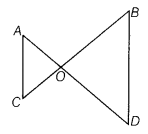# In the given figure, ∠AOC = ∠ACO and ∠DBO = ∠BOD. Show that AC || BD

In the given figure, ∠AOC = ∠ACO and ∠DBO = ∠BOD. Show that AC || BD.Given ∠AOC = ∠ACO and ∠DBO = ∠BOD
To prove AC||BD
Proof∠AOC =∠ACO [given]
∠AOC = ∠BOD
[vertically opposite angles]
∠ACO = ∠BOD ,…(i)
∠BOD=∠DBO [given]…(ii) (1)
From Eqs. (i) and (ii), we get
∠ACO =∠DBO
∴ AC || BD [∵ alternate interior angles are equal]